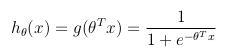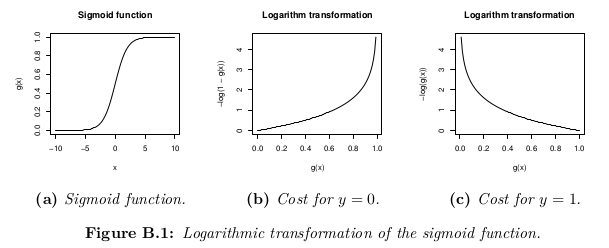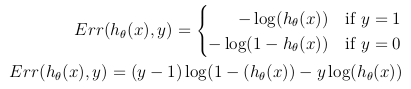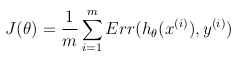2 views

cost function for the logistic regression is

cost(h(theta)X,Y) = -log(h(theta)X) or -log(1-h(theta)X)

My question is what is the base of putting the logarithmic expression for the cost function. Where does it come from? I believe you can't just put "-log" out of nowhere. If someone could explain the derivation of the cost function I would be grateful. thank you.

by (33.1k points)

## Hypothesis function

Logistic regression is used for classification tasks, that predict discrete values as a result.

If you consider a binary classification problem, then the hypothesis function is bounded between [0, 1]

Logistic regression formula:## Cost function

The cost function represents the optimization objective.The cost function could be the mean of the Euclidean distance between the hypothesis h_θ(x) and the actual value y among all them samples in the training set, when the hypothesis function is formed using the sigmoid function, this term would result in a non-convex cost function, which means that a local minimum can be easily located before reaching the global minimum. The cost function is convex, it is transformed using the logarithm of the sigmoid function.In this way, the optimization objective function can be defined as the mean of the costs/errors in the training set: